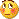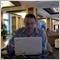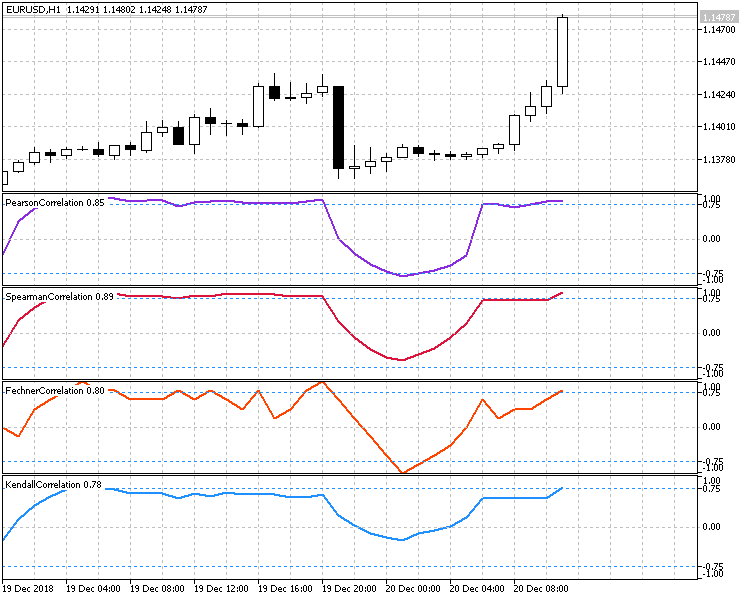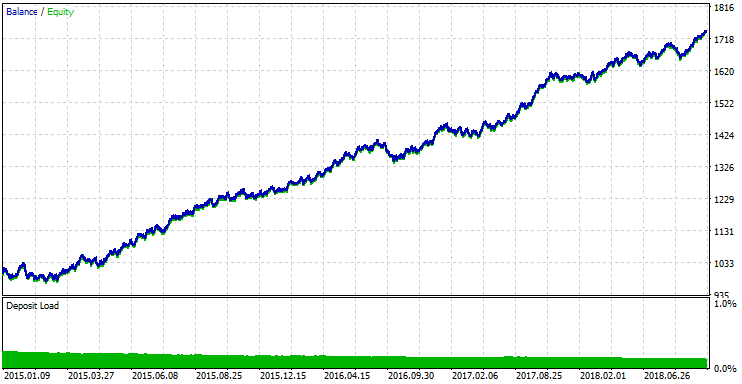# Correlation Index help!113

Daos anybody here who can say me why in the hell this indicator won't work?

Thank you

Files:610

albedo:
Daos anybody here who can say me why in the hell this indicator won't work? Thank you

U have to problems:

1) the definition of GbpUsd was not an Array.

it was

2) U have zero divizion

Change it to :

double tmp = MathSqrt((sumX2-(MathPow(sumX,2)/R_Period))*(sumY2-(MathPow(sumY,2)/R_Period)));

if (tmp == 0) tmp = Point;

R[acc]=(sumXY-((sumX*sumY)/R_Period))/ tmp;

Files:113

elihayun:
U have to problems:

1) the definition of GbpUsd was not an Array.

it was

2) U have zero divizion

Change it to :

double tmp = MathSqrt((sumX2-(MathPow(sumX,2)/R_Period))*(sumY2-(MathPow(sumY,2)/R_Period)));

if (tmp == 0) tmp = Point;

R[acc]=(sumXY-((sumX*sumY)/R_Period))/ tmp;

Oh, i see... thank you

however, now i can see its value, the problem is that this value should be between 1 and -1 and with this solution it shows values greater than 1

i have to give a deep look to the Pearson formula...

Thank you again..

maybe, you want to check the Pearson formula....... no, eh?10

thx. i was looking for it

albedo:
Oh, i see... thank you

however, now i can see its value, the problem is that this value should be between 1 and -1 and with this solution it shows values greater than 1

i have to give a deep look to the Pearson formula...

Thank you again..

maybe, you want to check the Pearson formula....... no, eh?Moderator
120153

Sergey Golubev, 2019.03.06 17:27

Good article was published -

----------------Correlation is a statistical relationship between two or more random variables (or quantities which can be considered random with some acceptable degree of accuracy). Changes in one ore more variables lead to systematic changes of other related variables. The mathematical measure of the correlation of two random variables is the correlation coefficient. If a change in one random variable does not lead to a regular change in the other random variable but leads to a change in another statistical characteristic of this random variable, such a relation is not considered correlation, although it is statistical.